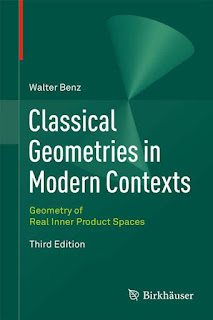GEOMETRY MATHEMATICS

# Classical Geometries in Modern Contexts Geometry of Real Inner Product Spaces 3rd Edition PDF Free DownloadThe focus of this book and its geometric notions is on real vector spaces X that are finite or infinite inner product spaces of arbitrary dimension greater than or equal to 2. It characterizes both euclidean and hyperbolic geometry with respect to natural properties of (general) translations and general distances of X. Also for these spaces X, it studies the sphere geometries of Möbius and Lie as well as geometries where Lorentz transformations play the key role. Proofs of newer theorems characterizing isometries and Lorentz transformations under mild hypotheses are included, such as for instance infinite dimensional versions of famous theorems of A.D. Alexandrov on Lorentz transformations. A real benefit is the dimension-free approach to important geometrical theories.